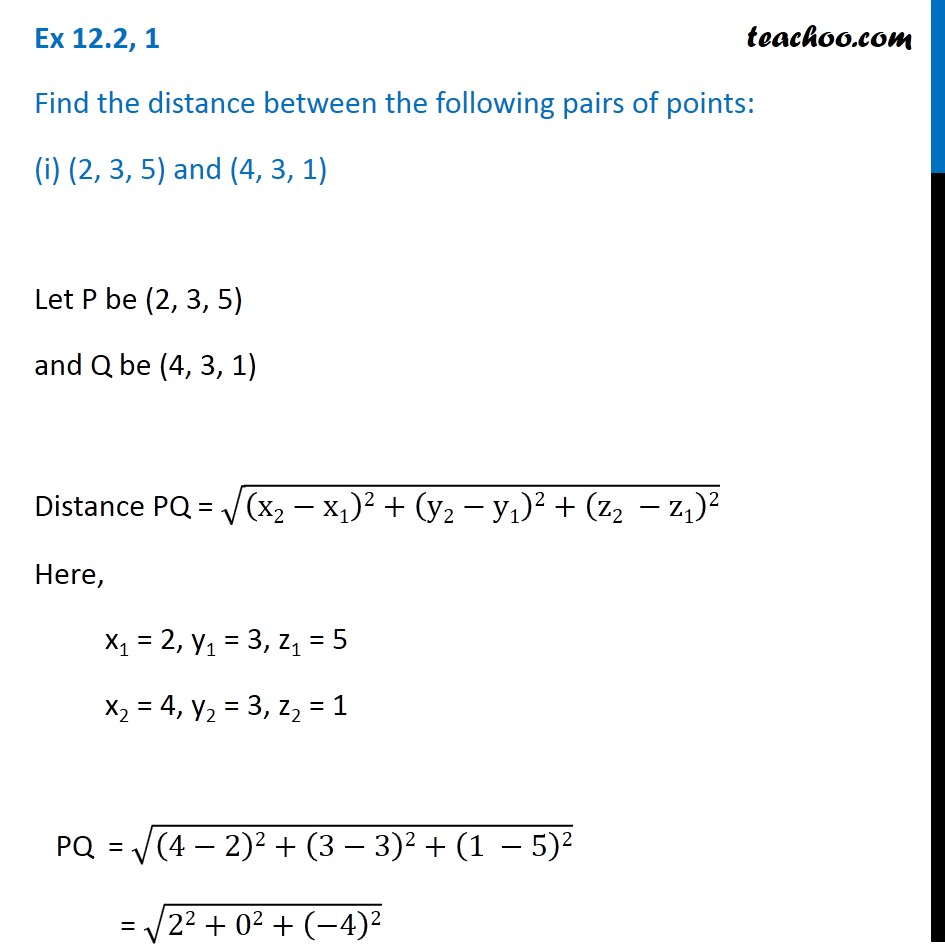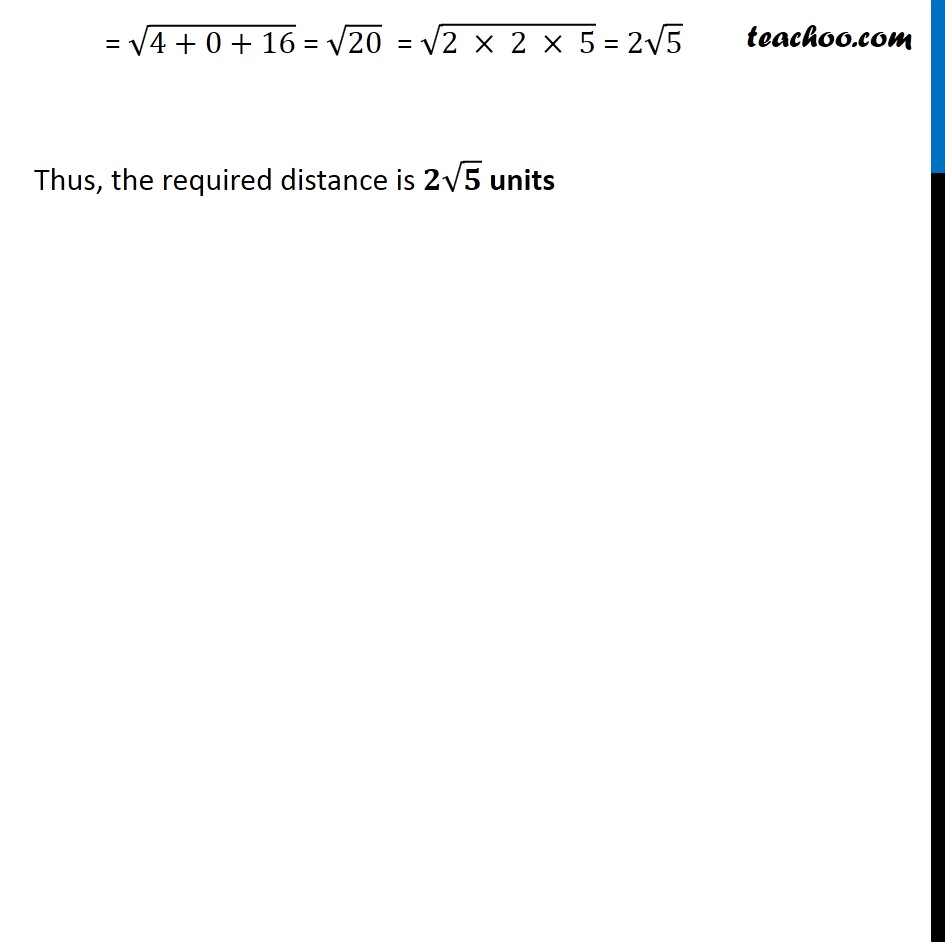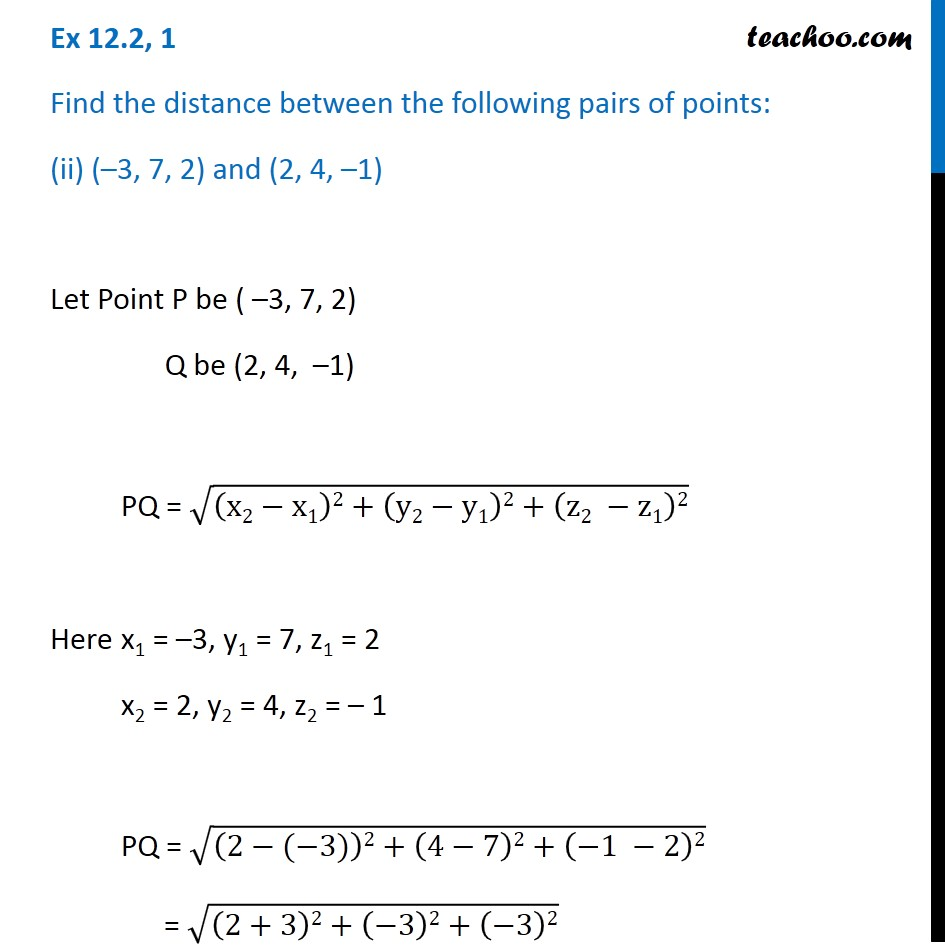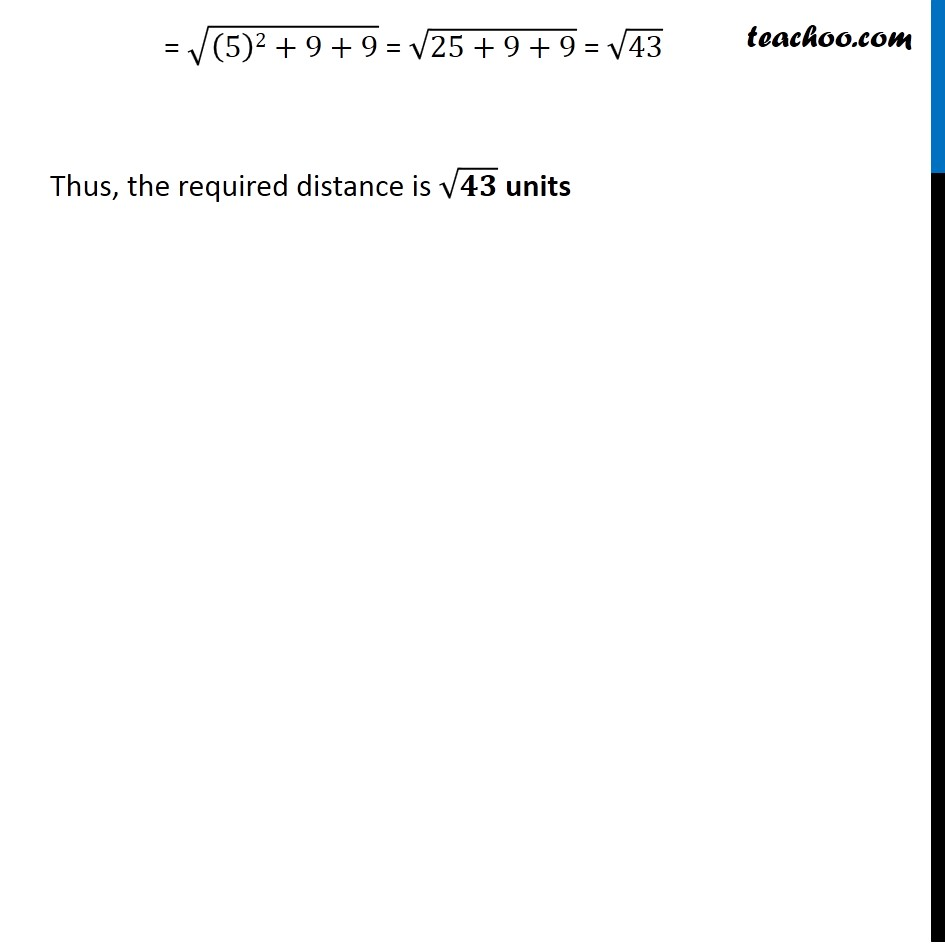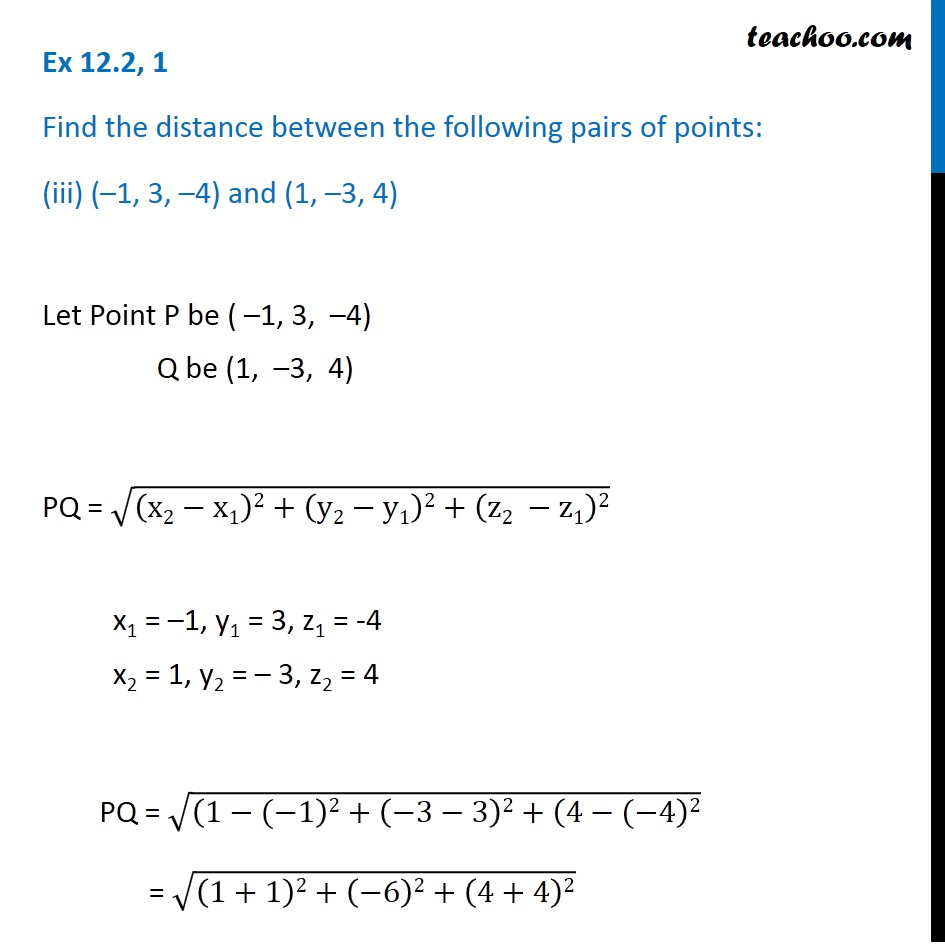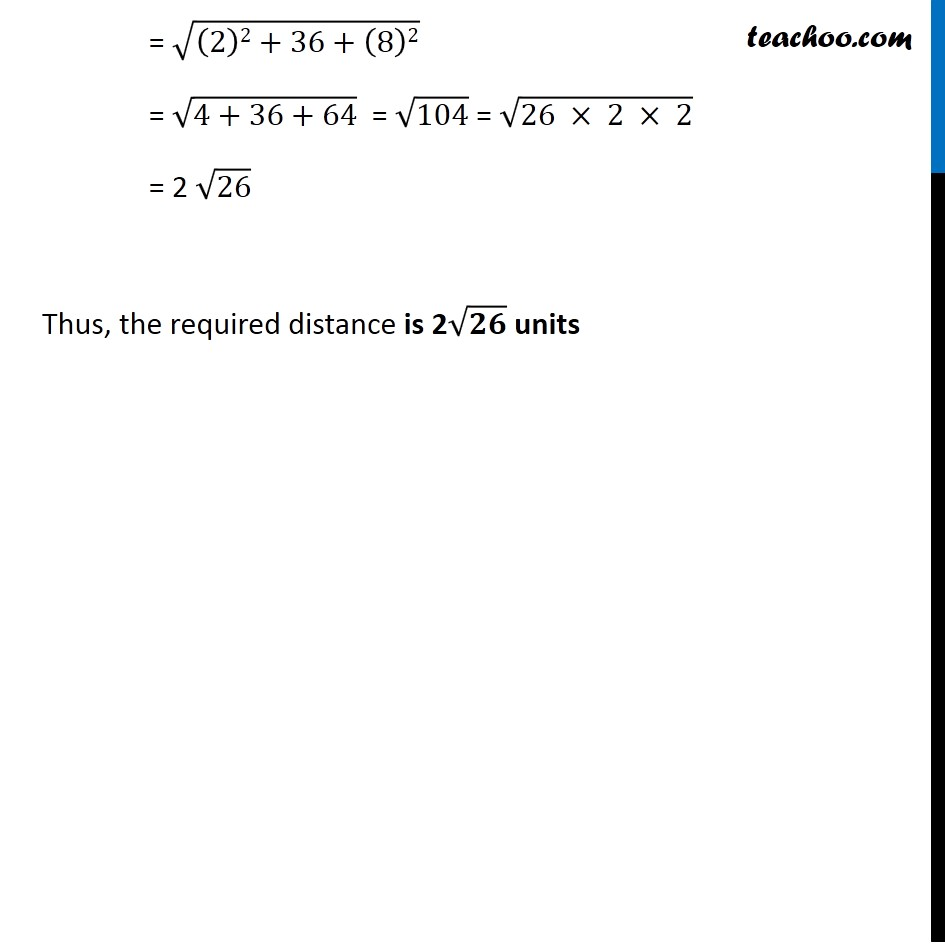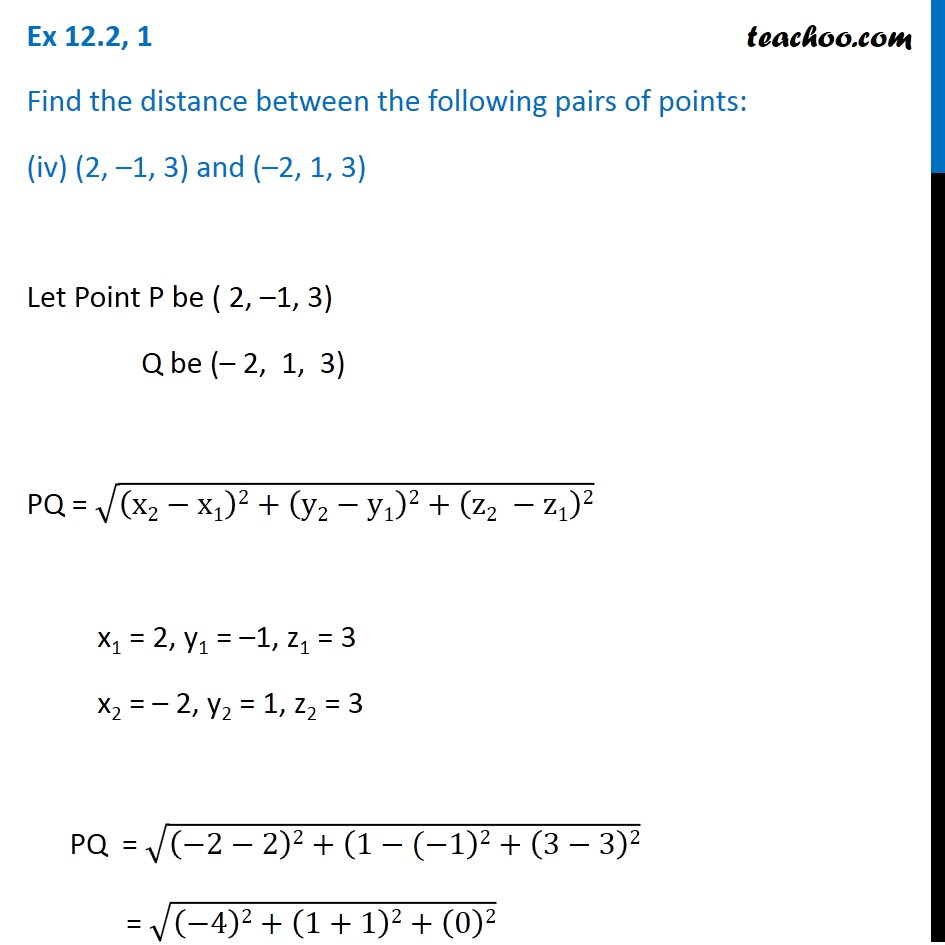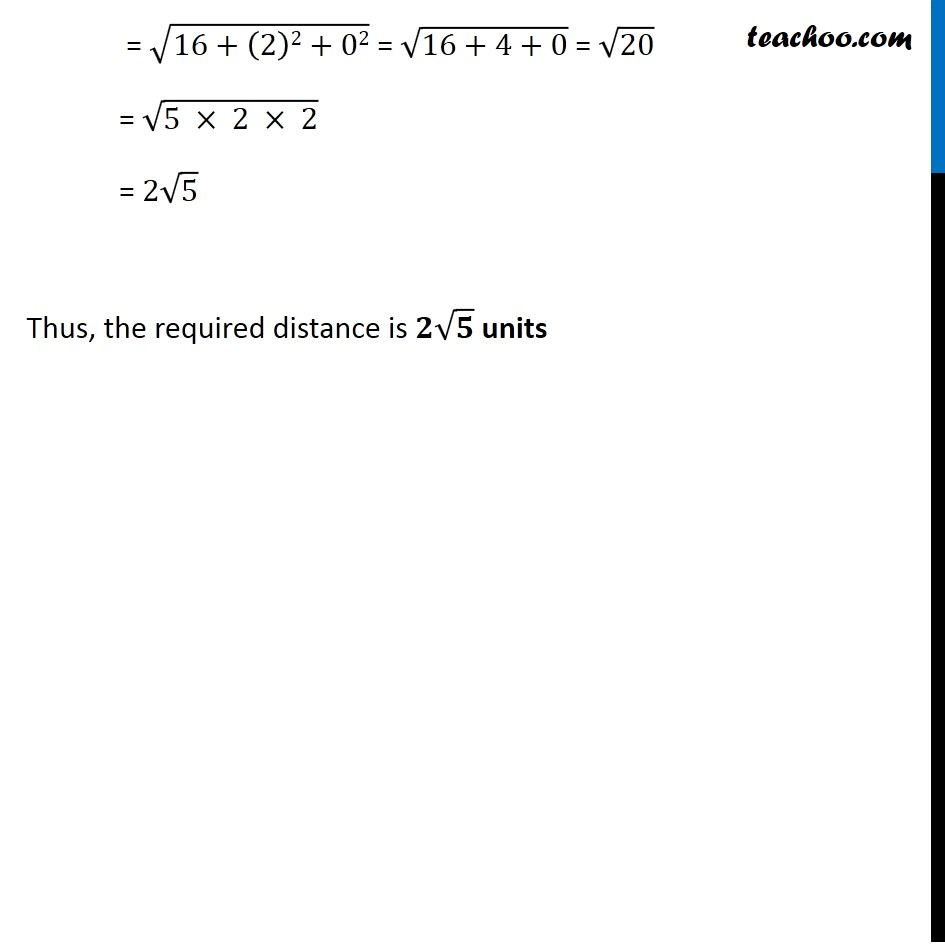Subscribe to our Youtube Channel - https://you.tube/teachoo

1. Chapter 12 Class 11 Introduction to Three Dimensional Geometry
2. Serial order wise
3. Ex 12.2

Transcript

Ex 12.2, 1 Find the distance between the following pairs of points: (i) (2, 3, 5) and (4, 3, 1) Let P be (2, 3, 5) and Q be (4, 3, 1) Distance PQ = √((x2−x1)2+(y2−y1)2+(z2 −z1)2) Here, x1 = 2, y1 = 3, z1 = 5 x2 = 4, y2 = 3, z2 = 1 PQ = √((4−2)2+(3−3)2+(1 −5)2) = √(22+02+(−4)2) = √(4+0+16) = √20 = √(2 ×2 ×5) = 2√5 Thus, the required distance is 2√5 units Ex 12.2, 1 Find the distance between the following pairs of points: (ii) (–3, 7, 2) and (2, 4, –1) Let Point P be ( –3, 7, 2) Q be (2, 4, –1) PQ = √((x2−x1)2+(y2−y1)2+(z2 −z1)2) Here x1 = –3, y1 = 7, z1 = 2 x2 = 2, y2 = 4, z2 = – 1 PQ = √((2−(−3))2+(4−7)2+(−1 −2)2) = √((2+3)2+(−3)2+(−3)2) = √((5)2+9+9) = √(25+9+9) = √43 Thus, the required distance is √𝟒𝟑 units Ex 12.2, 1 Find the distance between the following pairs of points: (iii) (–1, 3, –4) and (1, –3, 4) Let Point P be ( –1, 3, –4) Q be (1, –3, 4) PQ = √((x2−x1)2+(y2−y1)2+(z2 −z1)2) x1 = –1, y1 = 3, z1 = -4 x2 = 1, y2 = – 3, z2 = 4 PQ = √((1−(−1)2+(−3−3)2+(4−(−4)2) = √((1+1)2+(−6)2+(4+4)2) = √((2)2+36+(8)2) = √(4+36+64) = √104 = √(26 × 2\ × 2) = 2 √26 Thus, the required distance is 2√𝟐𝟔 units Ex 12.2, 1 Find the distance between the following pairs of points: (iv) (2, –1, 3) and (–2, 1, 3) Let Point P be ( 2, –1, 3) Q be (– 2, 1, 3) PQ = √((x2−x1)2+(y2−y1)2+(z2 −z1)2) x1 = 2, y1 = –1, z1 = 3 x2 = – 2, y2 = 1, z2 = 3 PQ = √((−2−2)2+(1−(−1)2+(3−3)2) = √((−4)2+(1+1)2+(0)2) = √(16+(2)2+02) = √(16+4+0) = √20 = √(5×2×2) = 2√5 Thus, the required distance is 2√5 units = √(16+(2)2+02) = √(16+4+0) = √20 = √(5 × 2 × 2) = 2√5 Thus, the required distance is 𝟐√𝟓 units = √(16+(2)2+02) = √(16+4+0) = √20 = √(5 × 2 × 2) = 2√5 Thus, the required distance is 𝟐√𝟓 units = √(16+(2)2+02) = √(16+4+0) = √20 = √(5 × 2 × 2) = 2√5 Thus, the required distance is 𝟐√𝟓 units

Ex 12.2